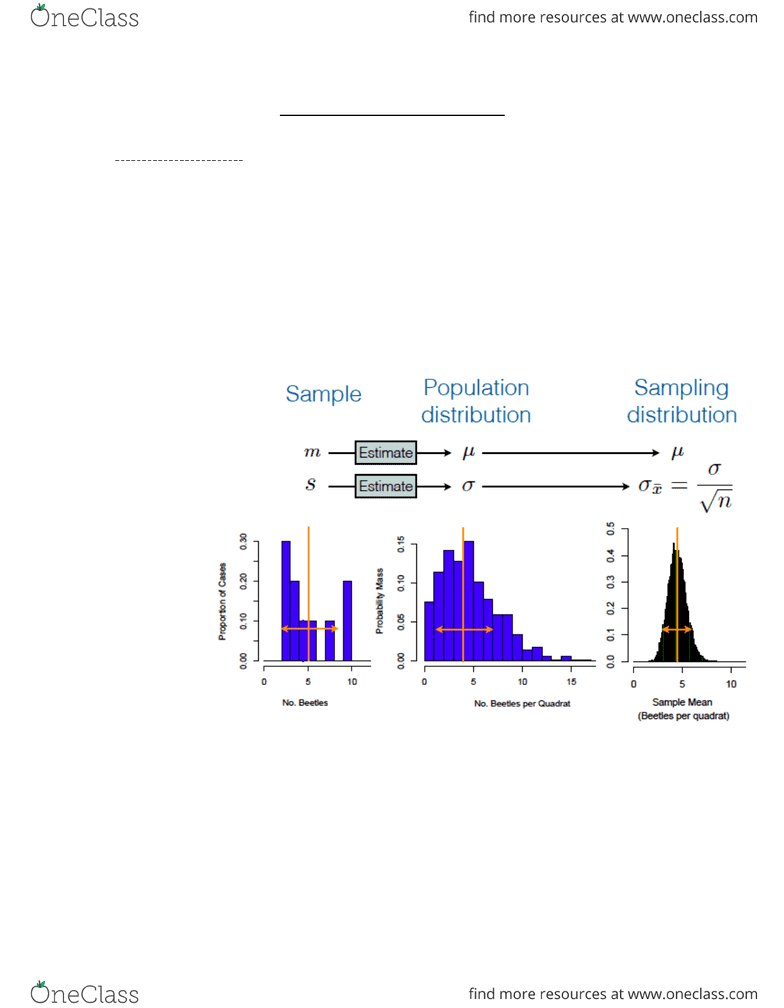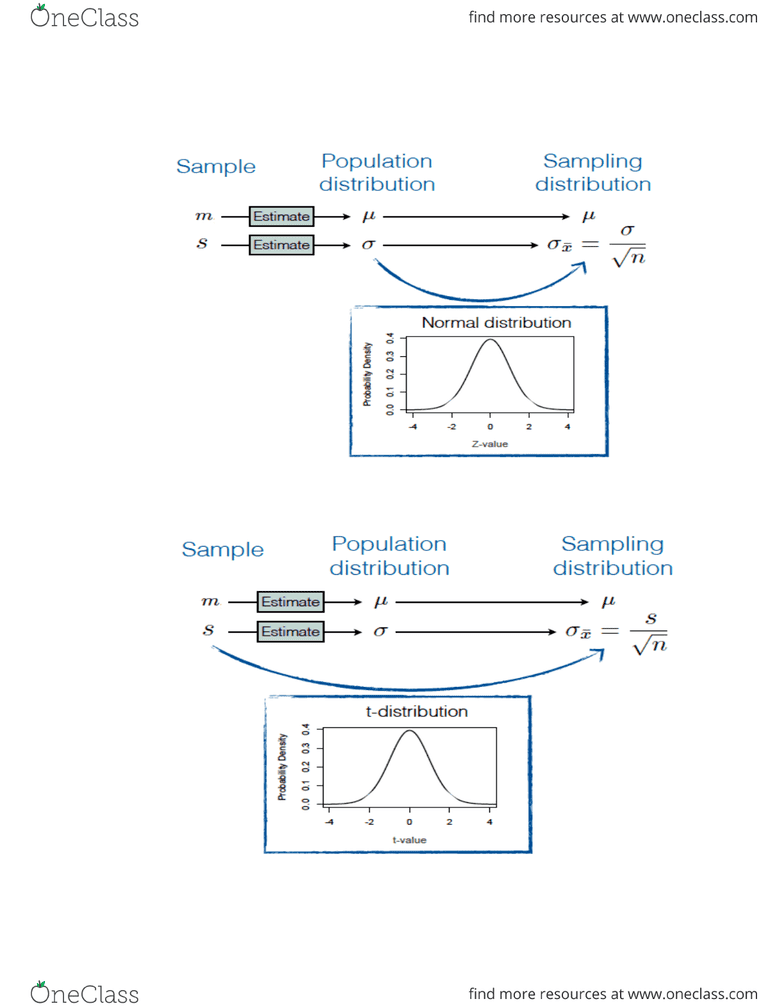Class Notes (1,100,000)
CA (620,000)
Queen's (10,000)
SOC (1,000)
Lecture 6

# SOCY 211 Lecture Notes - Lecture 6: Central Limit Theorem, Confidence Interval, Standard Deviation

Department
Social Sci, Edu and Soc Work - Sociology
Course Code
SOCY 211
Professor
Bill Nelson
Lecture
6

This preview shows pages 1-2. to view the full 6 pages of the document.SOCY 211 – Lecture 6
Module 5 Key ideas:
Sampling disstribution
Is the distribution of the sample mean.
A hypothetical distribution
Central limit theorem & Standard error
If we know the standard deviation of the sampling distribution, we
could use the normal distribution to represent the sample.
However, we can only estimate this, because it does not take the
uncertainty of standard deviation. Therefore, we use the t-
distribution.
If two people take different samples, they will have different
results, meaning they also have different population distributions.
The
μ
cannot change when choosing different samples
σ
is the standard deviation
If we know the standard deviation of the population distribution,
we can find the standard deviation of the sampling distribution.

Only pages 1-2 are available for preview. Some parts have been intentionally blurred.Normal distribution:
T-distribution:
###### You're Reading a Preview

Unlock to view full version